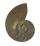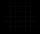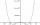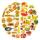# Exponentiation + real numbers - math problems

#### Number of problems found: 6

• Logarithmic equationSolve equation: log13(7x + 12) = 0
• Is equalIs equal following terms? -9 21 = (-9) 21By introducing a new variable solve biquadratic equation: ?
• EventsEvent P has probability of 0.84. What is the probability that the event P occurs in 3, 5, 7 try.
• CrystalThe crystal grows every month 1.2 permille of its mass. For how many months to grow a crystal from weight 177 g to 384 g?
• PoolIf water flows into the pool by two inlets, fill the whole for 19 hours. The first inlet filled pool 5 hour longer than the second. How long pool take to fill with two inlets separately?

We apologize, but in this category are not a lot of examples.
Do you have an interesting mathematical word problem that you can't solve it? Submit a math problem, and we can try to solve it.

We will send a solution to your e-mail address. Solved examples are also published here. Please enter the e-mail correctly and check whether you don't have a full mailbox.

Please do not submit problems from current active competitions such as Mathematical Olympiad, correspondence seminars etc...

Exponentiation - math problems. Real numbers - math problems.